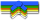# Search Results

Searching for: arrays

## Individual Exercises

0 / 50

Given an array containing three ints, return the sum of all the elements.0 / 50

Given two `int` arrays `a` and `b`, each with two elements, return a new array with four elements containing all the elements of `a` followed by...0 / 50

Given an `int` array of any length, return a new array of its first 2 elements. If the array is smaller than length 2, use whatever...0 / 50

Write a function in Java that takes an `int` array as its parameter and returns the number of even `int`s it contains. Note: the `%` "mod"...0 / 50

Write a function in Java that takes an `int` array as its parameter. It should return the sum of the numbers in the array, except ignore...0 / 50

We'll say that a value is "everywhere" in an array if for every pair of adjacent elements in the array, at least one of the pair is that...0 / 50

Given two arrays of the same length, `nums1` and `nums2`, for every element in `nums1`, consider the corresponding element in `nums2` (at the...0 / 50

Write a function in Java that returns a version of the given array where all the 10's have been removed. The remaining elements should...0 / 50

Write a function in Java that takes an array and returns the sum of the numbers in the array, or 0 if the array is empty. Except the...0 / 50

Given an array of `int` values, return true if the array contains either 3 even or 3 odd values all next to each other.0 / 50

For each multiple of 10 in the given array, change all the values following it to be that multiple of 10, until encountering another...0 / 70

Given an integer array length of 1 or more, return the difference between the largest and smallest values in the array. Note that the...0 / 70

Return the centered average of an array of `int`s, which we'll say is the mean average of the values, except ignoring the largest and...0 / 70

Given a number `n`, create and return a new `int` array of length `n`, containing the numbers 0, 1, 2, ... n-1. The given `n` may be 0, in which...0 / 70

Given a number `n`, create and return a new string array of length `n`, containing the strings "0", "1" "2" .. through n-1. `N` may be 0, in...0 / 70

Given an array of `int`s, return `true` if the array contains a 2 next to a 2 somewhere.0 / 70

Given an array of `int`s, return `true` if the array does not contain any 1s or 3s. Otherwise, return `false`.0 / 70

Given an array of `int`s, return `true` if the sum of all the 2's in the array is exactly 8.0 / 70

Given an array of `int`s, return `true` if the number of 1s is greater than the number of 4s. Otherwise, return `false`.0 / 70

Given an array of ints, return `true` if every element is either a 1 or a 4. Otherwise, return `false`.0 / 70

Consider the series of numbers beginning at `start` and running up to but not including `end`, so for example start=1 and end=5 gives the...0 / 70

Given an array of `int`s, if it contains no 1's return `true`. If it contains no 4's, return `true`. If it contains both, return `false`.0 / 70

Given an array of `int`s, return `true` if the array contains a 2 next to a 2 or a 4 next to a 4, but not both. Otherwise, return `false`.0 / 70

Given an array of `int`s, return `true` if the array contains two 7s next to each other, or there are two 7s separated by one element, such...0 / 70

Given an array of `int`s, return `true` if there is a 1 in the array with a 2 somewhere later in the array.